# Elbow Method for optimal value of k in KMeans

• Difficulty Level : Basic
• Last Updated : 18 May, 2022

Prerequisites: K-Means Clustering
A fundamental step for any unsupervised algorithm is to determine the optimal number of clusters into which the data may be clustered. The Elbow Method is one of the most popular methods to determine this optimal value of k.
We now demonstrate the given method using the K-Means clustering technique using the Sklearn library of python.Step 1: Importing the required libraries

## Python3

 `from` `sklearn.cluster ``import` `KMeans``from` `sklearn ``import` `metrics``from` `scipy.spatial.distance ``import` `cdist``import` `numpy as np``import` `matplotlib.pyplot as plt`

Step 2: Creating and Visualizing the data

## Python3

 `# Creating the data``x1 ``=` `np.array([``3``, ``1``, ``1``, ``2``, ``1``, ``6``, ``6``, ``6``, ``5``, ``6``, ``7``, ``8``, ``9``, ``8``, ``9``, ``9``, ``8``])``x2 ``=` `np.array([``5``, ``4``, ``5``, ``6``, ``5``, ``8``, ``6``, ``7``, ``6``, ``7``, ``1``, ``2``, ``1``, ``2``, ``3``, ``2``, ``3``])``X ``=` `np.array(``list``(``zip``(x1, x2))).reshape(``len``(x1), ``2``)`` ` `# Visualizing the data``plt.plot()``plt.xlim([``0``, ``10``])``plt.ylim([``0``, ``10``])``plt.title(``'Dataset'``)``plt.scatter(x1, x2)``plt.show()`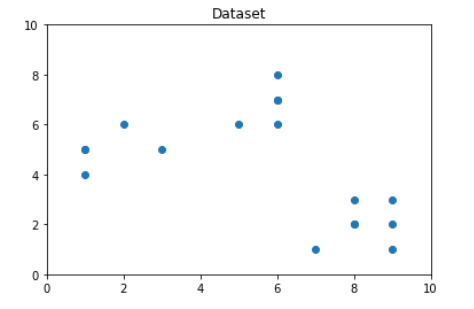From the above visualization, we can see that the optimal number of clusters should be around 3. But visualizing the data alone cannot always give the right answer. Hence we demonstrate the following steps.
We now define the following:-

1. Distortion: It is calculated as the average of the squared distances from the cluster centers of the respective clusters. Typically, the Euclidean distance metric is used.
2. Inertia: It is the sum of squared distances of samples to their closest cluster center.

We iterate the values of k from 1 to 9 and calculate the values of distortions for each value of k and calculate the distortion and inertia for each value of k in the given range.
Step 3: Building the clustering model and calculating the values of the Distortion and Inertia:

## Python3

 `distortions ``=` `[]``inertias ``=` `[]``mapping1 ``=` `{}``mapping2 ``=` `{}``K ``=` `range``(``1``, ``10``)`` ` `for` `k ``in` `K:``    ``# Building and fitting the model``    ``kmeanModel ``=` `KMeans(n_clusters``=``k).fit(X)``    ``kmeanModel.fit(X)`` ` `    ``distortions.append(``sum``(np.``min``(cdist(X, kmeanModel.cluster_centers_,``                                        ``'euclidean'``), axis``=``1``)) ``/` `X.shape[``0``])``    ``inertias.append(kmeanModel.inertia_)`` ` `    ``mapping1[k] ``=` `sum``(np.``min``(cdist(X, kmeanModel.cluster_centers_,``                                   ``'euclidean'``), axis``=``1``)) ``/` `X.shape[``0``]``    ``mapping2[k] ``=` `kmeanModel.inertia_`

Step 4: Tabulating and Visualizing the results
a) Using the different values of Distortion:

## Python3

 `for` `key, val ``in` `mapping1.items():``    ``print``(f``'{key} : {val}'``)`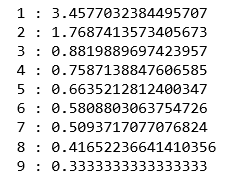## Python3

 `plt.plot(K, distortions, ``'bx-'``)``plt.xlabel(``'Values of K'``)``plt.ylabel(``'Distortion'``)``plt.title(``'The Elbow Method using Distortion'``)``plt.show()`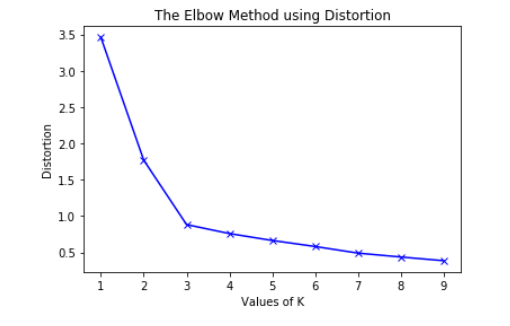b) Using the different values of Inertia:

## Python3

 `for` `key, val ``in` `mapping2.items():``    ``print``(f``'{key} : {val}'``)`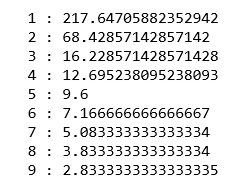## Python3

 `plt.plot(K, inertias, ``'bx-'``)``plt.xlabel(``'Values of K'``)``plt.ylabel(``'Inertia'``)``plt.title(``'The Elbow Method using Inertia'``)``plt.show()`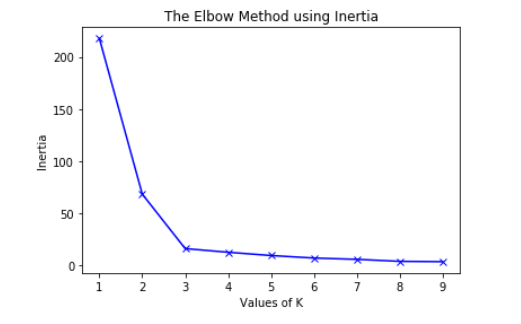To determine the optimal number of clusters, we have to select the value of k at the “elbow” ie the point after which the distortion/inertia start decreasing in a linear fashion. Thus for the given data, we conclude that the optimal number of clusters for the data is 3.
The clustered data points for different value of k:-
1. k = 1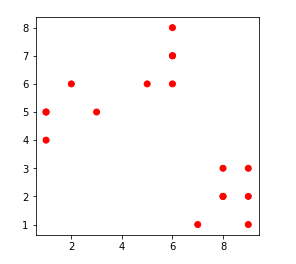2. k = 2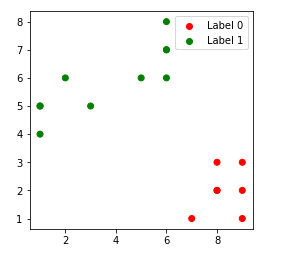3. k = 3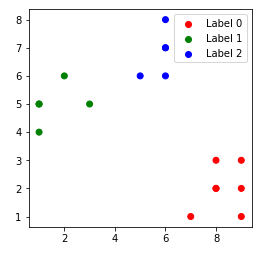4. k = 4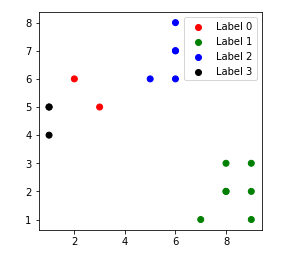My Personal Notes arrow_drop_up